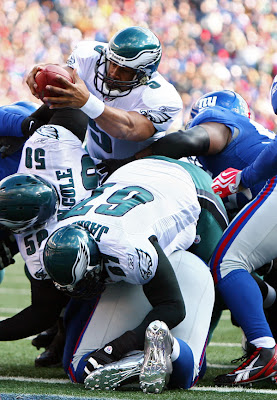## Wednesday, January 14, 2009

### 2008 Divisional Playoff GamesNet Total Off=Rushing+Drives Def=Rushing+Pressure

Pitt … 1692.23 … 894.50 = 622.00 + 272.50 … 797.73 = 501.88 + 295.85
SD … -684.78 … -165.75 = -40.75 + -125.00 … -519.03 = -501.88 + -17.16

Arz … 1135.39 … 656.80 = 355.50 + 301.30 … 478.59 = 285.63 + 192.96
Car … -167.65 … 45.70 = 204.50 + -158.80 … -213.35 = -285.63 + 72.27

Tenn … 821.18 … 580.90 = 308.00 + 272.90 … 240.28 = 136.25 + 104.03
Bal … 22.27 … -17.15 = 135.75 + -152.90 … 39.42 = -136.25 + 175.67

Phi … 440.25 … 329.00 = 216.00 + 113.00 … 111.25 = 19.38 + 91.88
NYG … 346.63 … 317.25 = 312.75 + 4.50 … 29.38 = -19.38 + 48.75